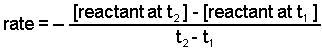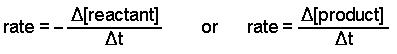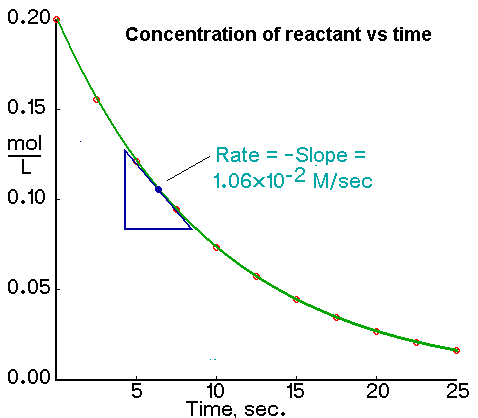Determining Reaction Rates

The rate of a reaction is expressed three ways:

Determining the Average Rate from Change in Concentration over a Time Period

We calculate the average rate of a reaction over a time interval by dividing the change in concentration over that time period by the time interval. For the change in concentration of a reactant, the equation, where the brackets mean "concentration of", isNote: We use the minus sign before the ratio in the previous equation because a rate is a positive number. We do not need the minus sign when calculating average rates from products.Top

Determining the Instantaneous Rate from a Plot of Concentration Versus Time

An instantaneous rate is the rate at some instant in time. An instantaneous rate is a differential rate: -d[reactant]/dt or d[product]/dt.

We determine an instantaneous rate at time t:

• by calculating the negative of the slope of the curve of concentration of a reactant versus time at time t.
• by calculating the slope of the curve of concentration of a product versus time at time t.Top

Determining the Initial Rate from a Plot of Concentration Versus Time

The initial rate of a reaction is the instantaneous rate at the start of the reaction (i.e., when t = 0). The initial rate is equal to the negative of the slope of the curve of reactant concentration versus time at t = 0.

Top